# 基数排序

## 排序原理

### 计数排序

（假设有无序序列：[ 5, 8, 9, 1, 4, 2, 9, 3, 7, 1, 8, 6, 2, 3, 4, 0, 8 ]）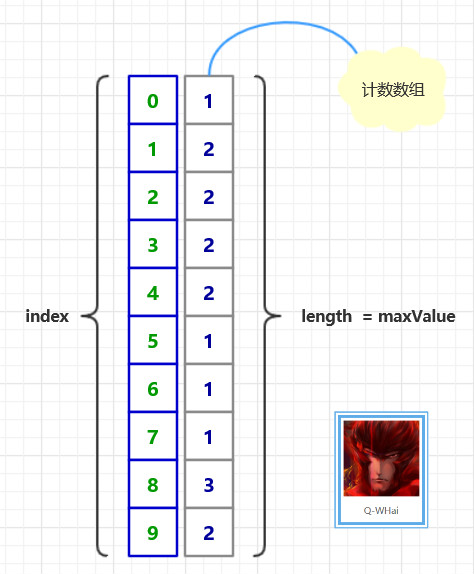### 基数排序原理图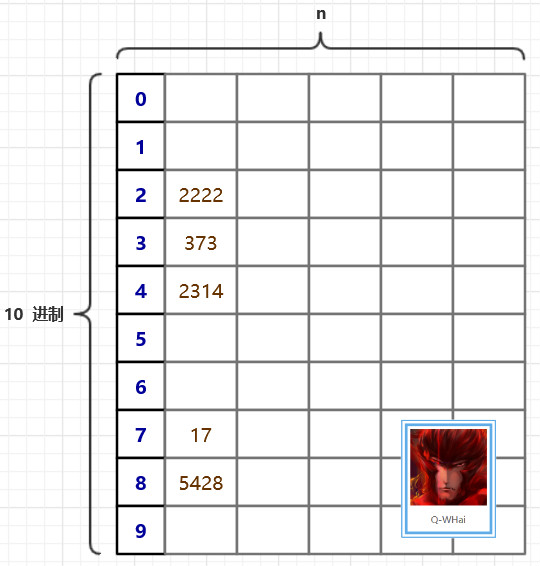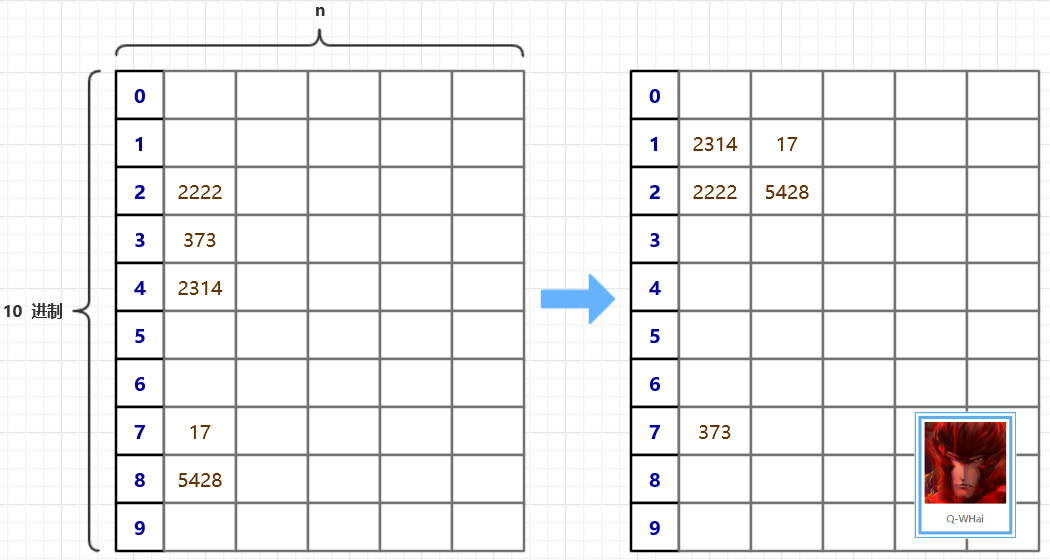## 算法优化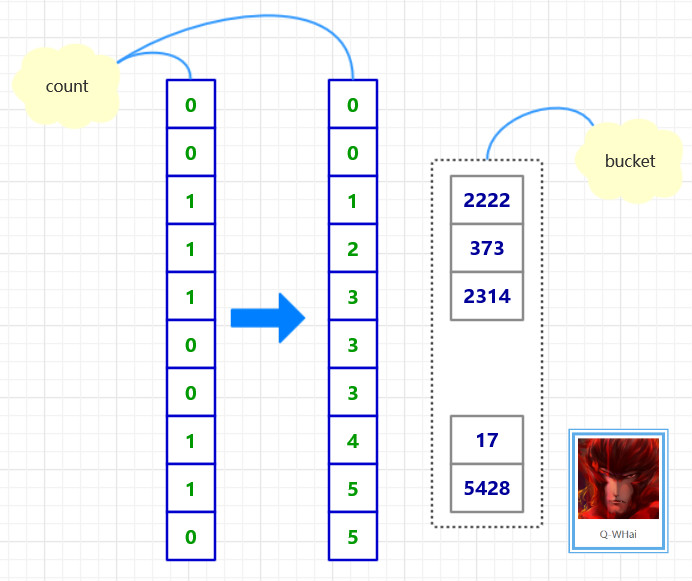## 算法实现

import org.algorithm.array.sort.interf.Sortable;

/**
* <p>
* 基数排序/桶排序
* </p>
* 2016年1月19日
*
* @author <a href="http://weibo.com/u/5131020927">Q-WHai</a>
* @see <a href="http://blog.csdn.net/lemon_tree12138">http://blog.csdn.net/lemon_tree12138</a>
* @version 0.1.1
*/
public class RadixSort implements Sortable {

@Override
public int[] sort(int[] array) {
if (array == null) {
return null;
}

int maxLength = maxLength(array);

return sortCore(array, 0, maxLength);
}

private int[] sortCore(int[] array, int digit, int maxLength) {
if (digit >= maxLength) {
return array;
}

final int radix = 10; // 基数
int arrayLength = array.length;
int[] count = new int[radix];
int[] bucket = new int[arrayLength];

// 统计将数组中的数字分配到桶中后，各个桶中的数字个数
for (int i = 0; i < arrayLength; i++) {
count[getDigit(array[i], digit)]++;
}

// 将各个桶中的数字个数，转化成各个桶中最后一个数字的下标索引
for (int i = 1; i < radix; i++) {
count[i] = count[i] + count[i - 1];
}

// 将原数组中的数字分配给辅助数组 bucket
for (int i = arrayLength - 1; i >= 0; i--) {
int number = array[i];
int d = getDigit(number, digit);
bucket[count[d] - 1] = number;
count[d]--;
}

return sortCore(bucket, digit + 1, maxLength);
}

/*
* 一个数组中最大数字的位数
*
* @param array
* @return
*/
private int maxLength(int[] array) {
int maxLength = 0;
int arrayLength = array.length;
for (int i = 0; i < arrayLength; i++) {
int currentLength = length(array[i]);
if (maxLength < currentLength) {
maxLength = currentLength;
}
}

return maxLength;
}

/*
* 计算一个数字共有多少位
*
* @param number
* @return
*/
private int length(int number) {
return String.valueOf(number).length();
}

/*
* 获取 x 这个数的 d 位数上的数字
* 比如获取 123 的 0 位数,结果返回 3
*
* @param x
* @param d
* @return
*/
private int getDigit(int x, int d) {
int a[] = { 1, 10, 100, 1000, 10000, 100000, 1000000, 10000000, 100000000, 1000000000 };
return ((x / a[d]) % 10);
}
}


### 基数排序过程图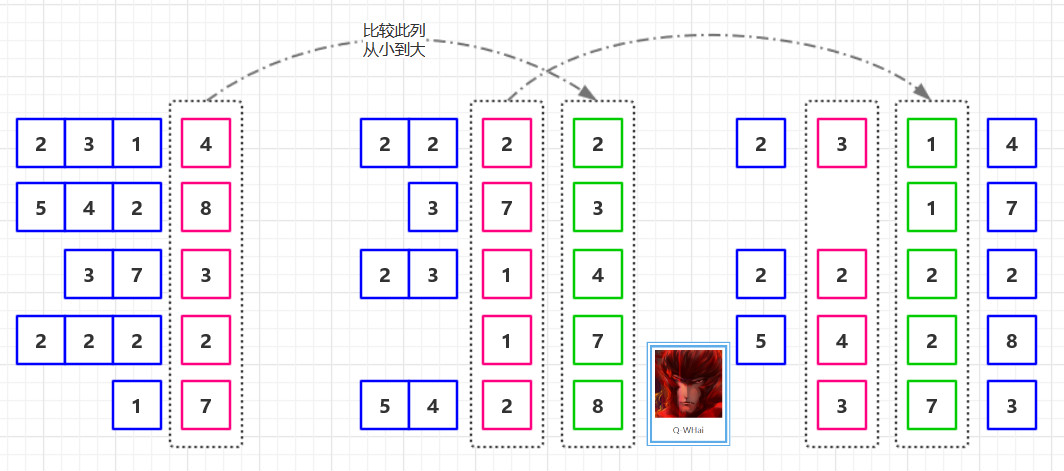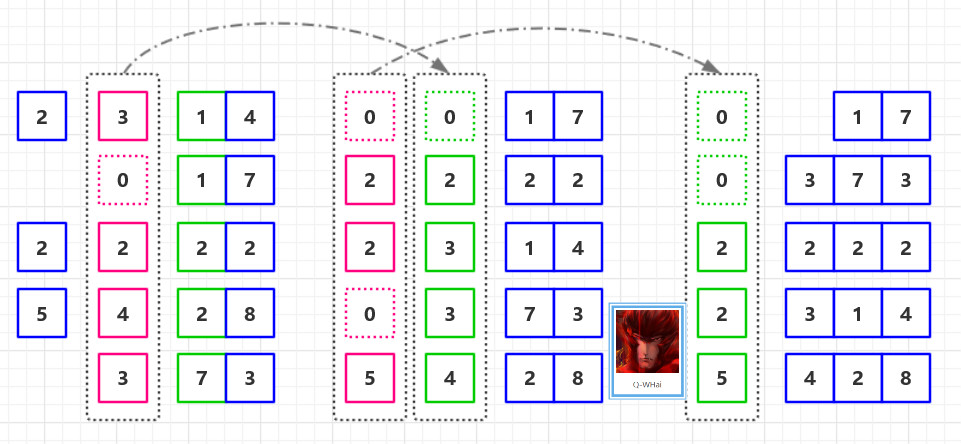## 复杂度分析

 排序方法 时间复杂度 空间复杂度 稳定性 复杂性 平均情况 最坏情况 最好情况 基数排序 O(d*(n+r)) O(d*(n+r)) O(d*(n+r)) O(n+r) 稳定 较复杂

• 《算法导论》

# GitHub Download

• https://github.com/qwhai/algorithms-sort

# 征集

https://www.processon.com/i/56205c2ee4b0f6ed10838a6d

10-0905-171万+
10-15104
02-091万+
02-147499
01-08
06-13767
12-03
07-15
12-08159
04-12
10-18
12-27
08-081万+
05-172万+
12-151万+
©️2020 CSDN 皮肤主题: 程序猿惹谁了 设计师:白松林